# UNIT 2 Darcy Law and Laboratory and Field

• Slides: 49UNIT 2 Darcy Law and Laboratory and Field Methods to determine aquifer propertiesDarcy Law � Henry Philibert Gaspard Darcy was French Engineer born on June 10, 1803 in Dijon, France. In 1856, carried out experiments while researching sand filters that lead to Darcy’s Law. � The law states that the flow rate (Q) through a porous medium is Ø directly proportion to the head loss, Ø directly proportional to the cross sectional area through which the water is flowing and Ø inversely proportional to the length of the flow path. � Darcy Law allows an estimate of the velocity or flow rate moving within the aquifer the average time of travel from the head of the aquifer to a point located downstream. � Darcy’s law provides an accurate description of the flow of ground water in almost all hydrogeologic environments.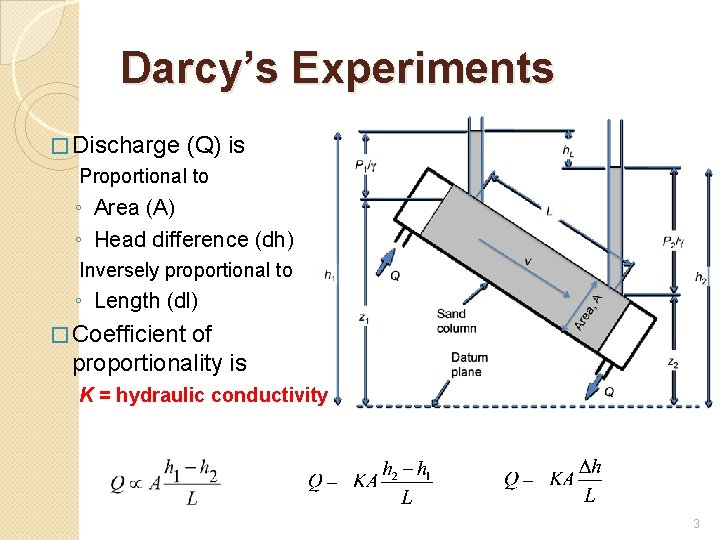Darcy’s Experiments � Discharge (Q) is Proportional to ◦ Area (A) ◦ Head difference (dh) Inversely proportional to ◦ Length (dl) � Coefficient of proportionality is K = hydraulic conductivity 3Darcy Law � Darcy measurements showed that the flow rate Q is directly proportional to the head loss (dh) and the cross-sectional area (A) of flow. Q α dh, Q α A � And inversely proportional to the length of the flow path Q α 1/dl � The above equations can be rearranged as Q α (dh*A)/dl � Introducing the proportionality constant K in the above equation we get the Darcy Law which can be written as Q = -K(dh*A)/dl or K*I*A � (I = dh/dl) The constant K is called hydraulic conductivity and depends both on the porous medium and on the fluid passing through it. The negative sign was introduced to obtain a positive value for the discharge; indeed, the groundwater flows in the direction of the decreasing head, which is negative.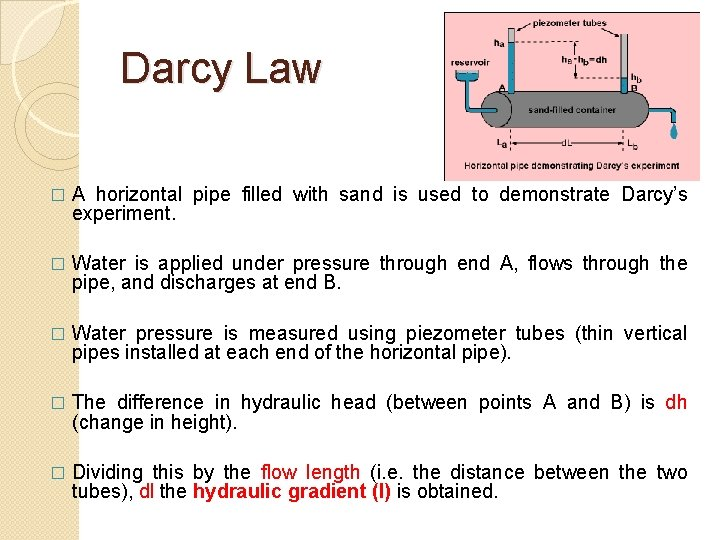Darcy Law � A horizontal pipe filled with sand is used to demonstrate Darcy’s experiment. � Water is applied under pressure through end A, flows through the pipe, and discharges at end B. � Water pressure is measured using piezometer tubes (thin vertical pipes installed at each end of the horizontal pipe). � The difference in hydraulic head (between points A and B) is dh (change in height). � Dividing this by the flow length (i. e. the distance between the two tubes), dl the hydraulic gradient (I) is obtained.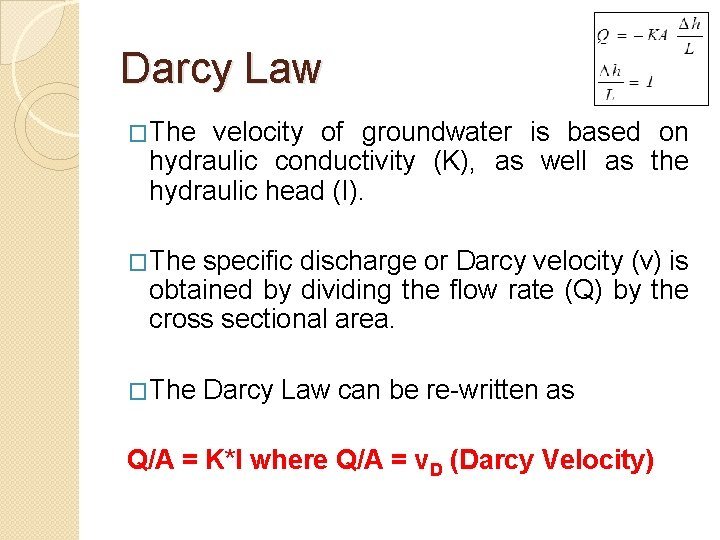Darcy Law �The velocity of groundwater is based on hydraulic conductivity (K), as well as the hydraulic head (I). �The specific discharge or Darcy velocity (v) is obtained by dividing the flow rate (Q) by the cross sectional area. �The Darcy Law can be re-written as Q/A = K*I where Q/A = v. D (Darcy Velocity)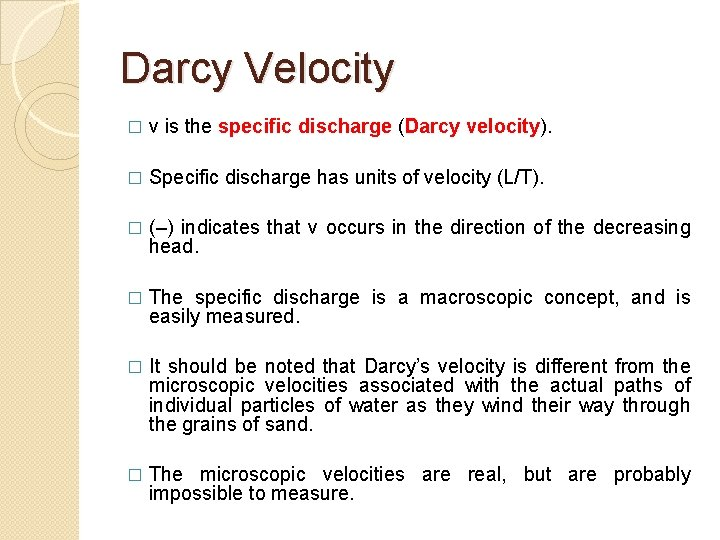Darcy Velocity � v is the specific discharge (Darcy velocity). � Specific discharge has units of velocity (L/T). � (–) indicates that v occurs in the direction of the decreasing head. � The specific discharge is a macroscopic concept, and is easily measured. � It should be noted that Darcy’s velocity is different from the microscopic velocities associated with the actual paths of individual particles of water as they wind their way through the grains of sand. � The microscopic velocities are real, but are probably impossible to measure.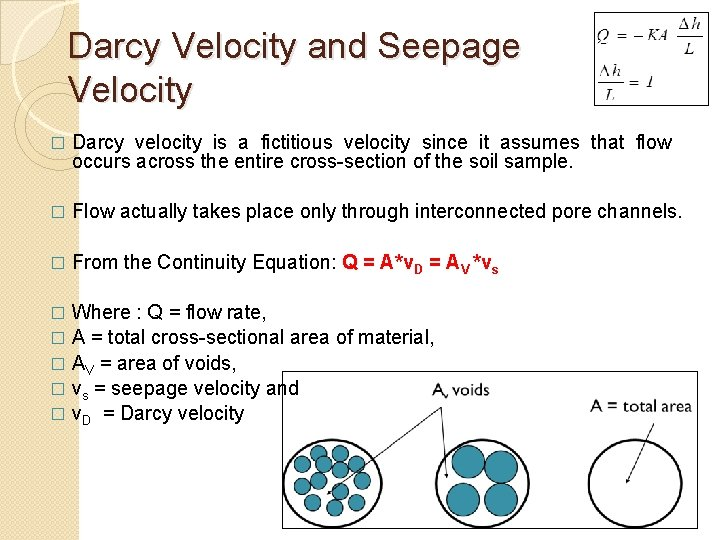Darcy Velocity and Seepage Velocity � Darcy velocity is a fictitious velocity since it assumes that flow occurs across the entire cross-section of the soil sample. � Flow actually takes place only through interconnected pore channels. � From the Continuity Equation: Q = A*v. D = AV *vs Where : Q = flow rate, � A = total cross-sectional area of material, � AV = area of voids, � vs = seepage velocity and � v. D = Darcy velocity �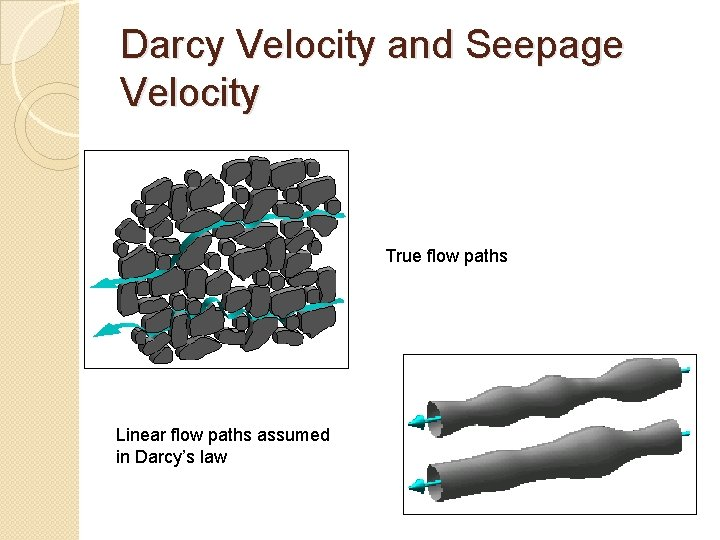Darcy Velocity and Seepage Velocity True flow paths Linear flow paths assumed in Darcy’s law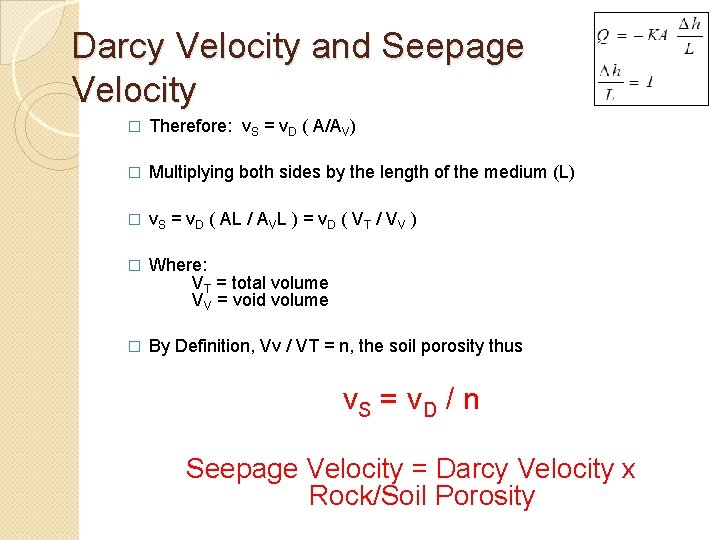Darcy Velocity and Seepage Velocity � Therefore: v. S = v. D ( A/AV) � Multiplying both sides by the length of the medium (L) � v. S = v. D ( AL / AVL ) = v. D ( VT / VV ) � Where: VT = total volume VV = void volume � By Definition, Vv / VT = n, the soil porosity thus v. S = v. D / n Seepage Velocity = Darcy Velocity x Rock/Soil PorosityDarcy Law Example A confined aquifer has a source of recharge. K for the aquifer is 50 m/day. � The water level in two wells 1000 m apart is 55 m and 50 m respectively. � The average thickness of the aquifer is 30 m, and the average width of aquifer is 5 km. � Calculate the flow rate and the seepage velocity if the effective porosity is 0. 27. �Validity of Darcy’s Law � In applying Darcy’s law it is important to know the range of validity within which it is applicable. � For flow in pipes or other large sections, the Reynolds number which expresses the dimensionless ratio of inertial to viscous forces, serves as a criterion to distinguish between laminar and turbulent flow. � Reynolds number (NR) is expresses as NR = ρv. D/μ � Where ρ is the fluid density, v is the velocity, D is the diameter of the pipe and μ is the viscosity of the fluid. � Experiments show that Darcy’s law is valid for NR < 1 and does not depart seriously up to NR = 1 LAMINAR FLOW TURBULENT FLOW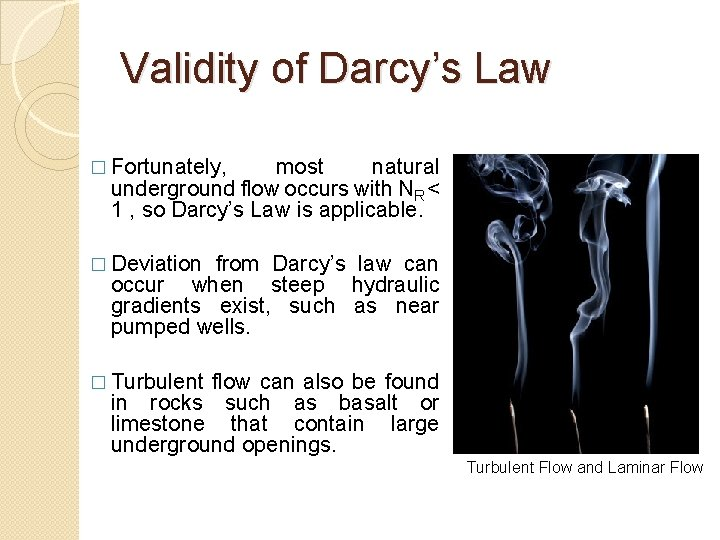Validity of Darcy’s Law � Fortunately, most natural underground flow occurs with NR < 1 , so Darcy’s Law is applicable. � Deviation from Darcy’s law can occur when steep hydraulic gradients exist, such as near pumped wells. � Turbulent flow can also be found in rocks such as basalt or limestone that contain large underground openings. Turbulent Flow and Laminar Flow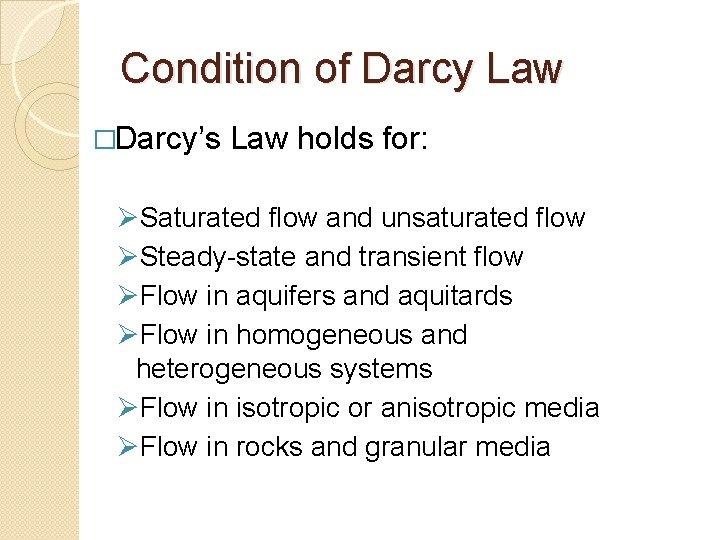Condition of Darcy Law �Darcy’s Law holds for: ØSaturated flow and unsaturated flow ØSteady-state and transient flow ØFlow in aquifers and aquitards ØFlow in homogeneous and heterogeneous systems ØFlow in isotropic or anisotropic media ØFlow in rocks and granular mediaPermeabilty � The permeability of a rock or soil defines its ability to transmit a fluid. Intrinsic Permeability � It is the portion of hydraulic conductivity which is representative of the properties of the porous medium alone (not the fluid) � It is a function of size of the openings through which the fluid moves k = C d 2 Where C = dimensionless constant, � d = mean pore diameter � � Dimensions of Intrinsic permeability is L 2 which is the same as Area. � Intrinsic permeability thus depends only on the property of the medium and not the fluid.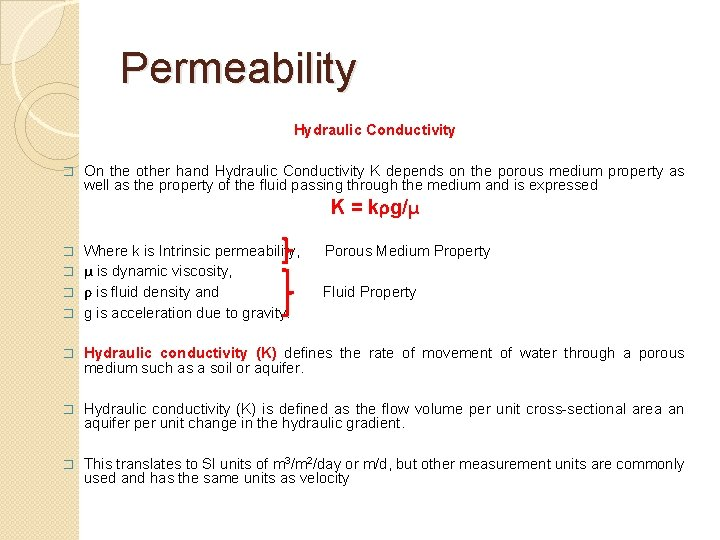Permeability Hydraulic Conductivity � On the other hand Hydraulic Conductivity K depends on the porous medium property as well as the property of the fluid passing through the medium and is expressed K = kρg/μ Where k is Intrinsic permeability, � μ is dynamic viscosity, � ρ is fluid density and � g is acceleration due to gravity. � Porous Medium Property Fluid Property � Hydraulic conductivity (K) defines the rate of movement of water through a porous medium such as a soil or aquifer. � Hydraulic conductivity (K) is defined as the flow volume per unit cross-sectional area an aquifer per unit change in the hydraulic gradient. � This translates to SI units of m 3/m 2/day or m/d, but other measurement units are commonly used and has the same units as velocity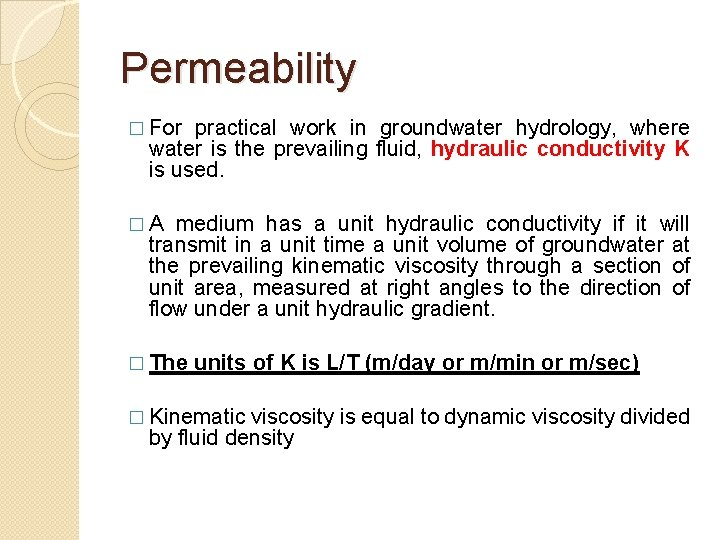Permeability � For practical work in groundwater hydrology, where water is the prevailing fluid, hydraulic conductivity K is used. �A medium has a unit hydraulic conductivity if it will transmit in a unit time a unit volume of groundwater at the prevailing kinematic viscosity through a section of unit area, measured at right angles to the direction of flow under a unit hydraulic gradient. � The units of K is L/T (m/day or m/min or m/sec) � Kinematic viscosity is equal to dynamic viscosity divided by fluid density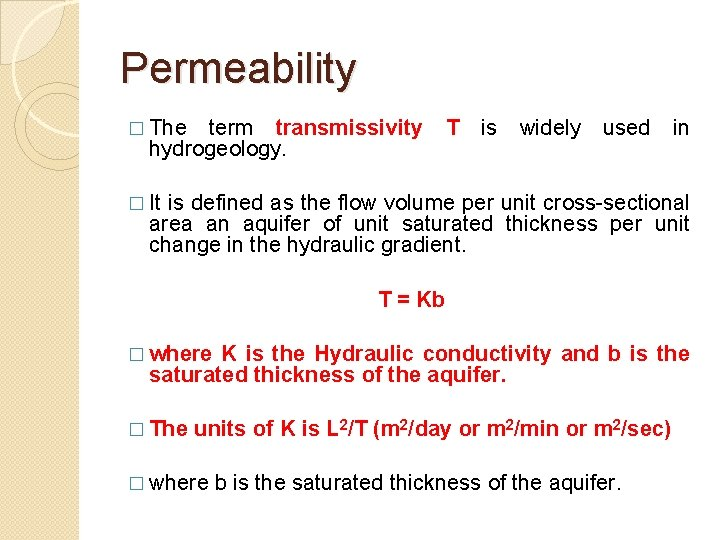Permeability � The term transmissivity hydrogeology. T is widely used in � It is defined as the flow volume per unit cross-sectional area an aquifer of unit saturated thickness per unit change in the hydraulic gradient. T = Kb � where K is the Hydraulic conductivity and b is the saturated thickness of the aquifer. � The units of K is L 2/T (m 2/day or m 2/min or m 2/sec) � where b is the saturated thickness of the aquifer.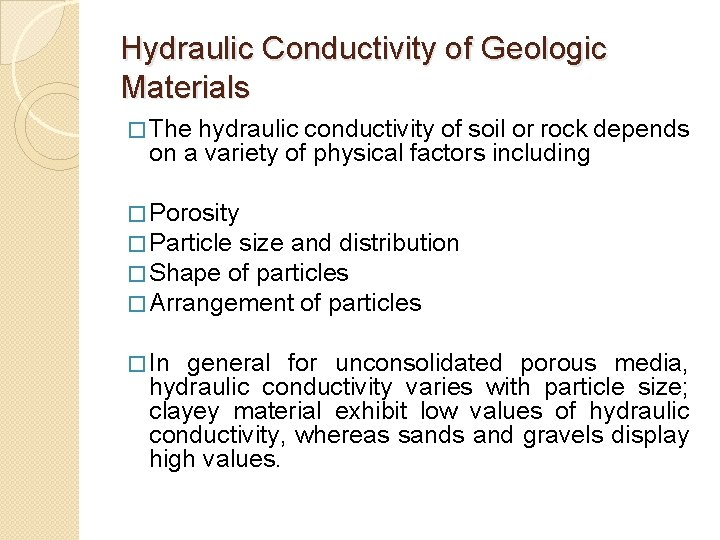Hydraulic Conductivity of Geologic Materials � The hydraulic conductivity of soil or rock depends on a variety of physical factors including � Porosity � Particle size and distribution � Shape of particles � Arrangement of particles � In general for unconsolidated porous media, hydraulic conductivity varies with particle size; clayey material exhibit low values of hydraulic conductivity, whereas sands and gravels display high values.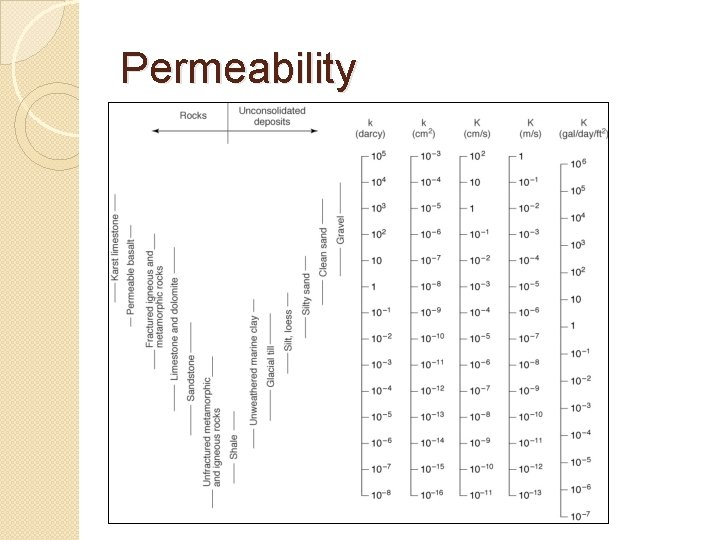Permeability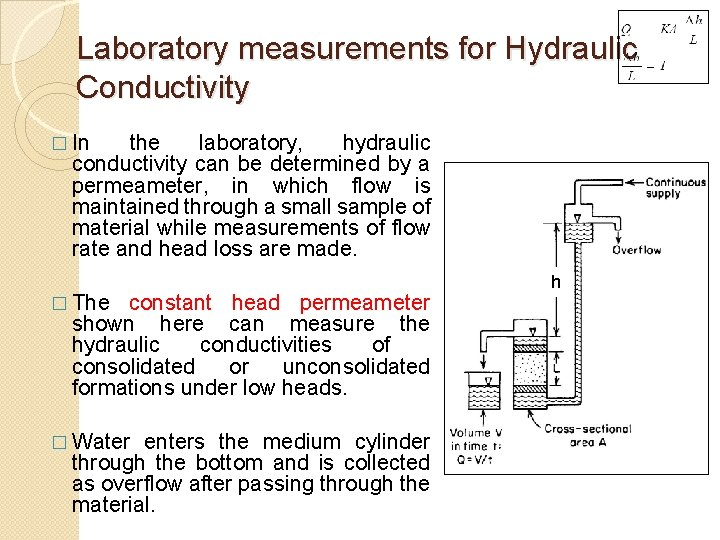Laboratory measurements for Hydraulic Conductivity � In the laboratory, hydraulic conductivity can be determined by a permeameter, in which flow is maintained through a small sample of material while measurements of flow rate and head loss are made. � The constant head permeameter shown here can measure the hydraulic conductivities of consolidated or unconsolidated formations under low heads. � Water enters the medium cylinder through the bottom and is collected as overflow after passing through the material. hLaboratory measurements for Hydraulic Conductivity � From Darcy’s law it follows that the hydraulic conductivity can be obtained from � Where V is the flow volume in time t and the other dimensions A, L and h are shown in the figure. � It is important to fully saturate the sample to remove entrapped air. � Several measurements at different heads in a series gives a reliable result of the K measurement. h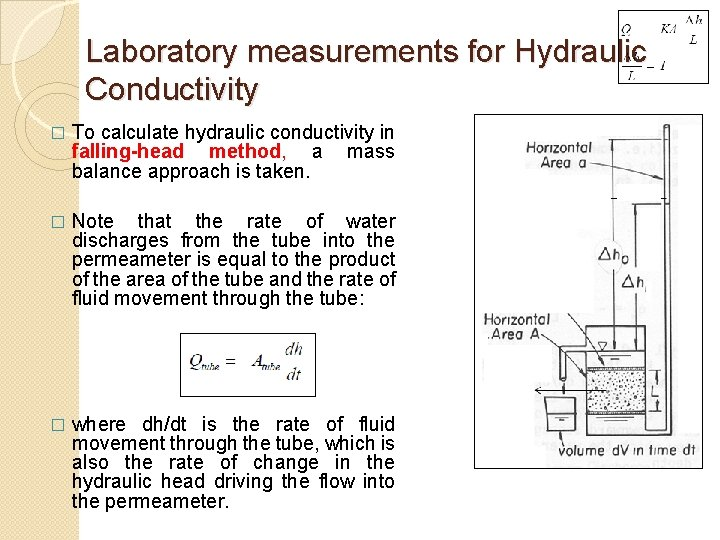Laboratory measurements for Hydraulic Conductivity � To calculate hydraulic conductivity in falling-head method, a mass balance approach is taken. � Note that the rate of water discharges from the tube into the permeameter is equal to the product of the area of the tube and the rate of fluid movement through the tube: � where dh/dt is the rate of fluid movement through the tube, which is also the rate of change in the hydraulic head driving the flow into the permeameter.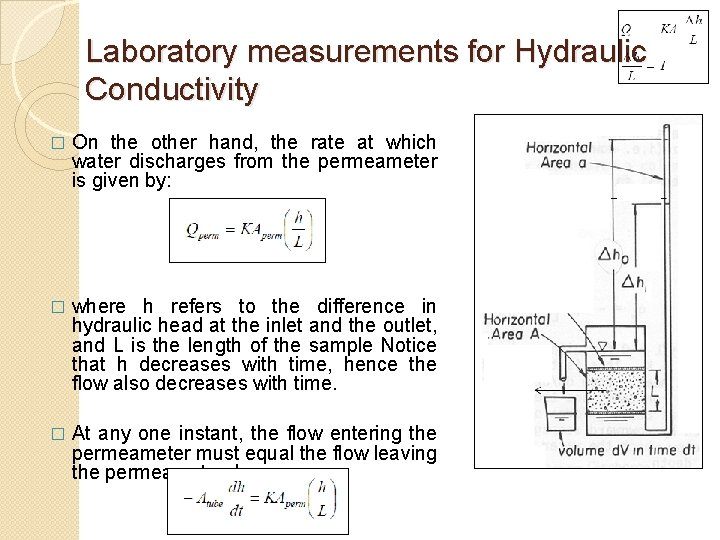Laboratory measurements for Hydraulic Conductivity � On the other hand, the rate at which water discharges from the permeameter is given by: � where h refers to the difference in hydraulic head at the inlet and the outlet, and L is the length of the sample Notice that h decreases with time, hence the flow also decreases with time. � At any one instant, the flow entering the permeameter must equal the flow leaving the permeameter, hence: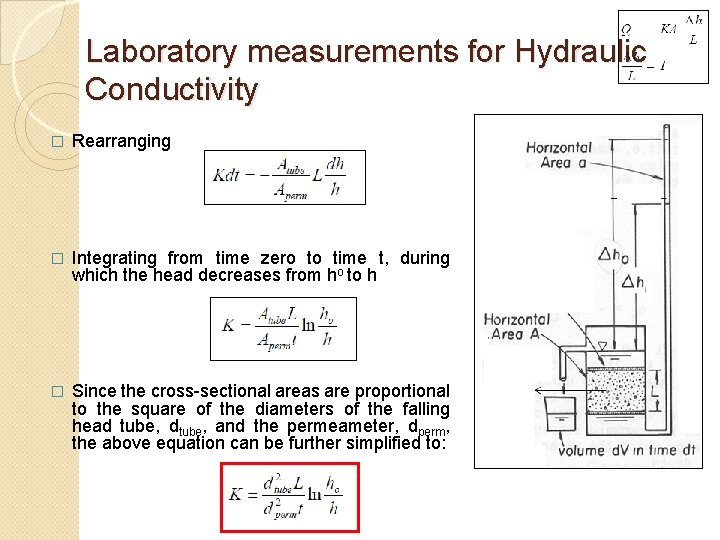Laboratory measurements for Hydraulic Conductivity � Rearranging � Integrating from time zero to time t, during which the head decreases from ho to h � Since the cross-sectional areas are proportional to the square of the diameters of the falling head tube, dtube, and the permeameter, dperm, the above equation can be further simplified to: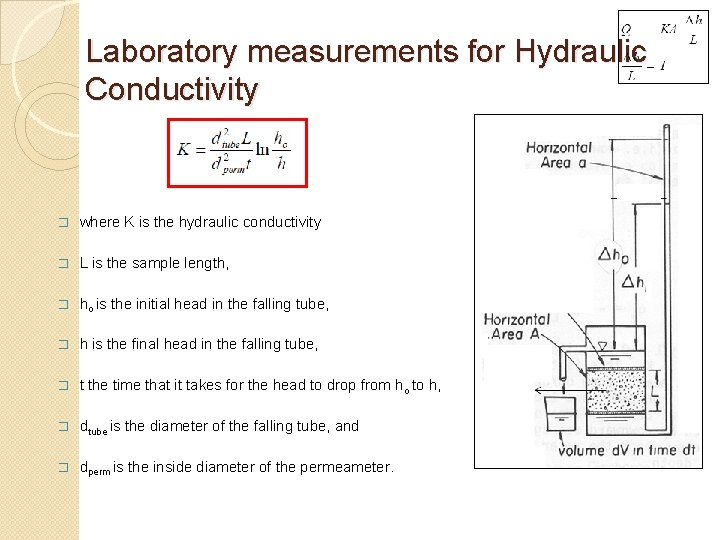Laboratory measurements for Hydraulic Conductivity � where K is the hydraulic conductivity � L is the sample length, � ho is the initial head in the falling tube, � h is the final head in the falling tube, � t the time that it takes for the head to drop from ho to h, � dtube is the diameter of the falling tube, and � dperm is the inside diameter of the permeameter.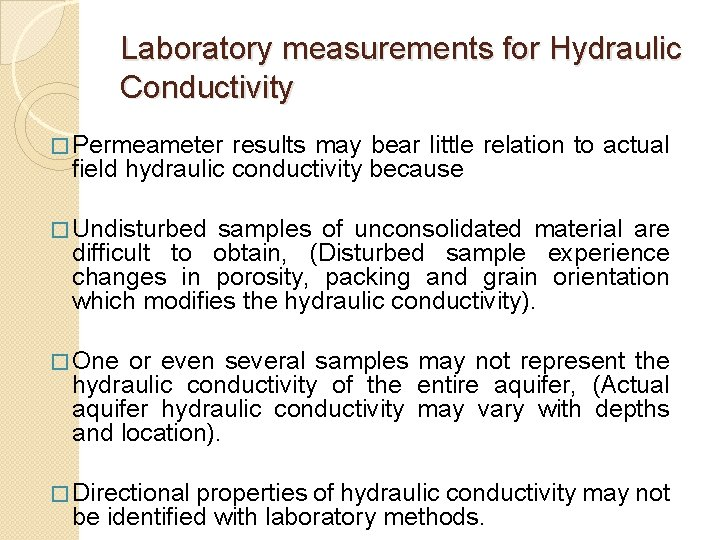Laboratory measurements for Hydraulic Conductivity � Permeameter results may bear little relation to actual field hydraulic conductivity because � Undisturbed samples of unconsolidated material are difficult to obtain, (Disturbed sample experience changes in porosity, packing and grain orientation which modifies the hydraulic conductivity). � One or even several samples may not represent the hydraulic conductivity of the entire aquifer, (Actual aquifer hydraulic conductivity may vary with depths and location). � Directional properties of hydraulic conductivity may not be identified with laboratory methods.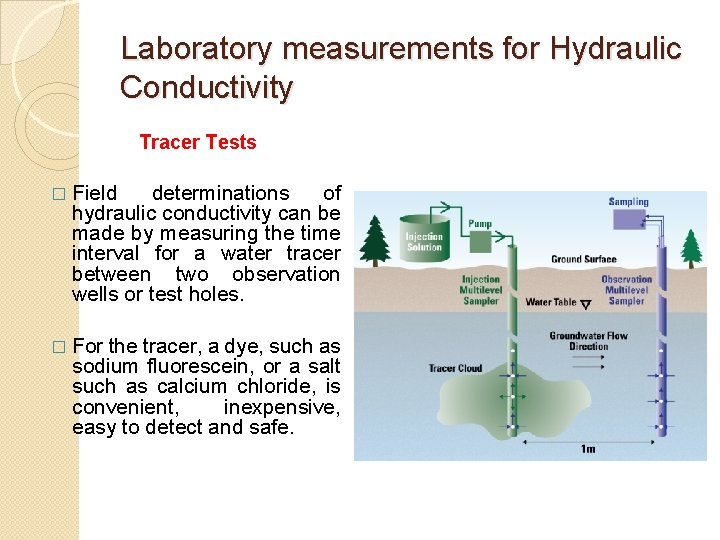Laboratory measurements for Hydraulic Conductivity Tracer Tests � Field determinations of hydraulic conductivity can be made by measuring the time interval for a water tracer between two observation wells or test holes. � For the tracer, a dye, such as sodium fluorescein, or a salt such as calcium chloride, is convenient, inexpensive, easy to detect and safe.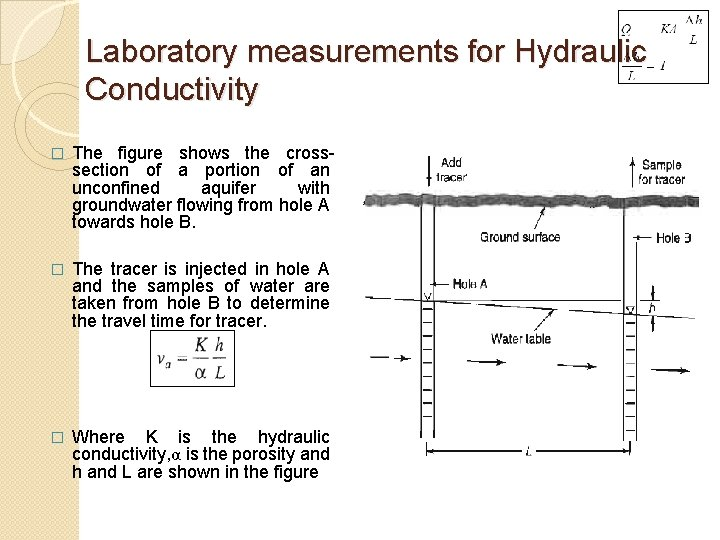Laboratory measurements for Hydraulic Conductivity � The figure shows the crosssection of a portion of an unconfined aquifer with groundwater flowing from hole A towards hole B. � The tracer is injected in hole A and the samples of water are taken from hole B to determine the travel time for tracer. � Where K is the hydraulic conductivity, α is the porosity and h and L are shown in the figure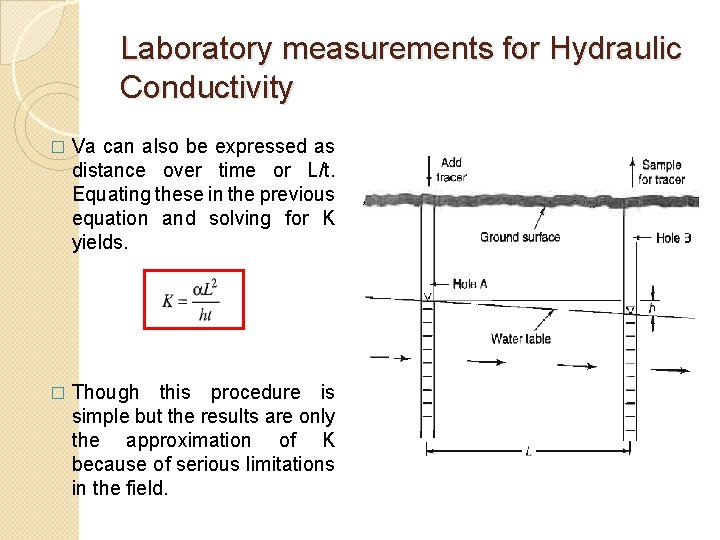Laboratory measurements for Hydraulic Conductivity � Va can also be expressed as distance over time or L/t. Equating these in the previous equation and solving for K yields. � Though this procedure is simple but the results are only the approximation of K because of serious limitations in the field.Laboratory measurements for Hydraulic Conductivity �Limitations of tracer tests are the following: �The holes need to be close together, otherwise the travel time interval can be excessively long. �Unless the flow direction is accurately known, the tracer may miss the downstream hole entirely. �If the aquifer is layered with different hydraulic conductivities, the first arrival of the tracer will result in a conductivity considerably larger than the average of the aquifer.Laboratory measurements for Hydraulic Conductivity � A tracer test is conducted to determine the hydraulic conductivity of an unconfined aquifer. The water levels in the two observation wells 20 m apart are 18. 4 m and 17. 1 m respectively. The tracer injected in the first well reaches the second well in 167 hours. Calculate the hydraulic conductivity of the unconfined aquifer if the porosity of the formation is 0. 25.Laboratory measurements for Hydraulic Conductivity �The most reliable method for estimating aquifer hydraulic conductivity is by pumping test of wells. �Based on observation of water levels near pumping wells, an integrated K value over a big section of the aquifer can be obtained �The reliability of this method is much superior to the methods discussed earlier.Hydraulic Conductivity for Anisotropic Aquifer �All the pervious calculations of hydraulic conductivity was based on the assumption that the geologic material was homogeneous and isotropic, implying that the hydraulic conductivity was equal in all directions. �However, for undisturbed , unconsolidated alluvial material it is rarely the case.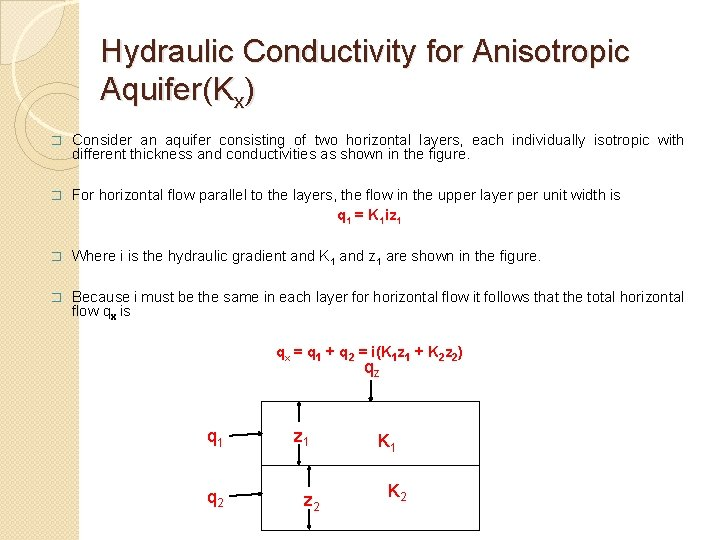Hydraulic Conductivity for Anisotropic Aquifer(Kx) � Consider an aquifer consisting of two horizontal layers, each individually isotropic with different thickness and conductivities as shown in the figure. � For horizontal flow parallel to the layers, the flow in the upper layer per unit width is q 1 = K 1 iz 1 � Where i is the hydraulic gradient and K 1 and z 1 are shown in the figure. � Because i must be the same in each layer for horizontal flow it follows that the total horizontal flow qx is qx = q 1 + q 2 = i(K 1 z 1 + K 2 z 2) qz q 1 q 2 z 1 z 2 K 1 K 2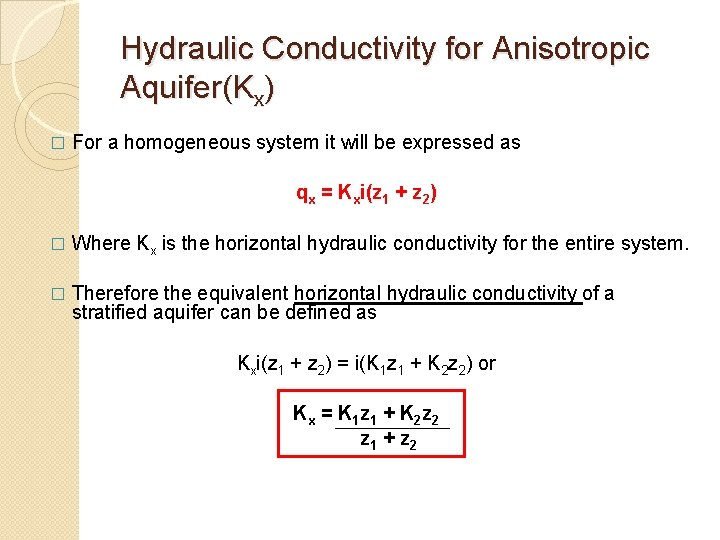Hydraulic Conductivity for Anisotropic Aquifer(Kx) � For a homogeneous system it will be expressed as qx = Kxi(z 1 + z 2) � Where Kx is the horizontal hydraulic conductivity for the entire system. � Therefore the equivalent horizontal hydraulic conductivity of a stratified aquifer can be defined as Kxi(z 1 + z 2) = i(K 1 z 1 + K 2 z 2) or Kx = K 1 z 1 + K 2 z 2 z 1 + z 2Hydraulic Conductivity for Anisotropic Aquifer(Kz) � Now for the vertical flow through the two layers in the figure � Where dh 1 is the head loss within the first layer. Solving for the head loss we get � From continuity equation, qz must be the same for the other layers so that the total head loss isHydraulic Conductivity for Anisotropic Aquifer(Kz) � For a homogeneous system � Where Kz is the vertical hydraulic conductivity for the entire system. Rearranging yields we get � Therefore equating with the equation for heterogeneous aquifer we get � This defines the vertical hydraulic conductivity for a stratified aquifer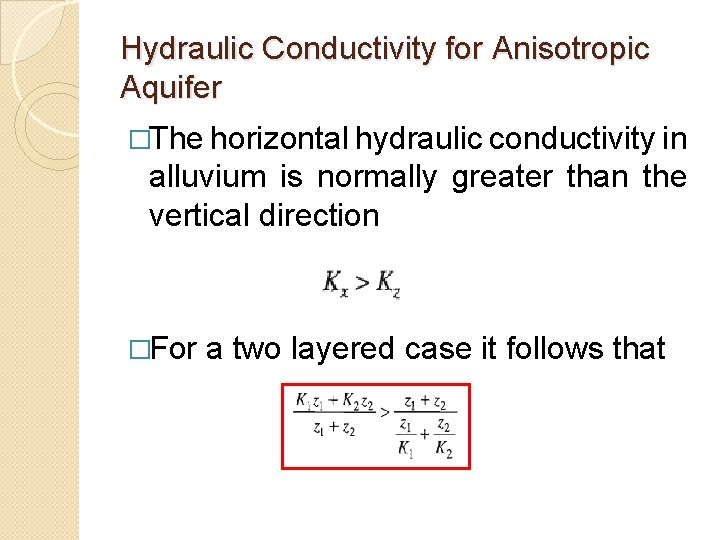Hydraulic Conductivity for Anisotropic Aquifer �The horizontal hydraulic conductivity in alluvium is normally greater than the vertical direction �For a two layered case it follows thatExample 40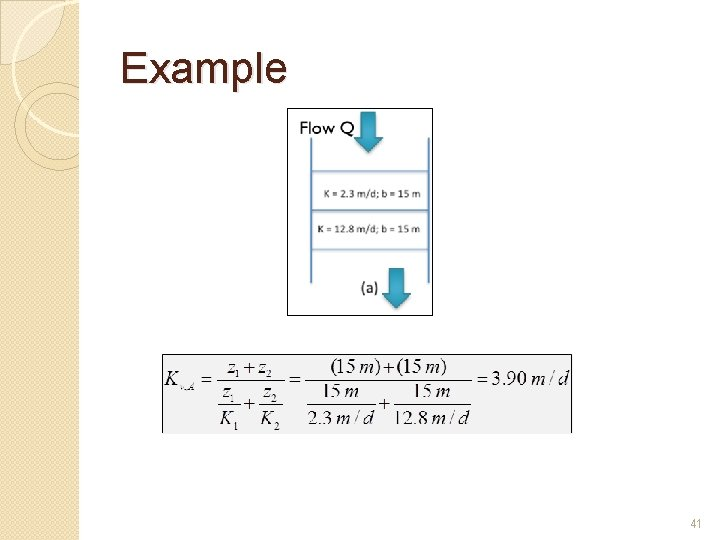Example 41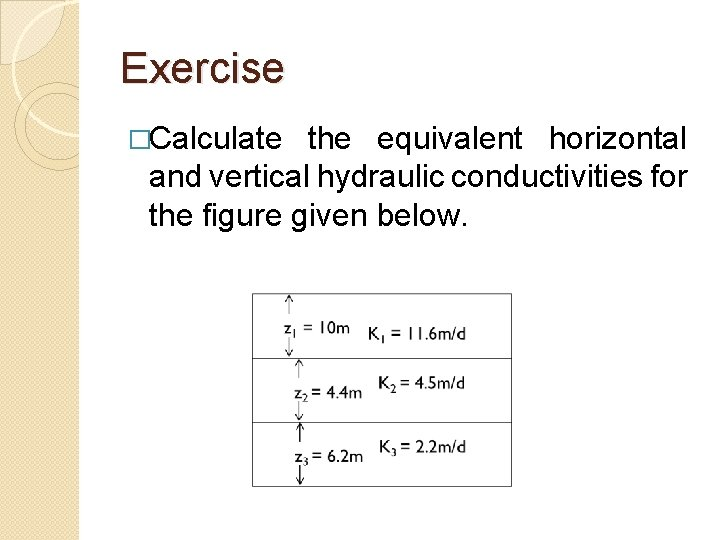Exercise �Calculate the equivalent horizontal and vertical hydraulic conductivities for the figure given below.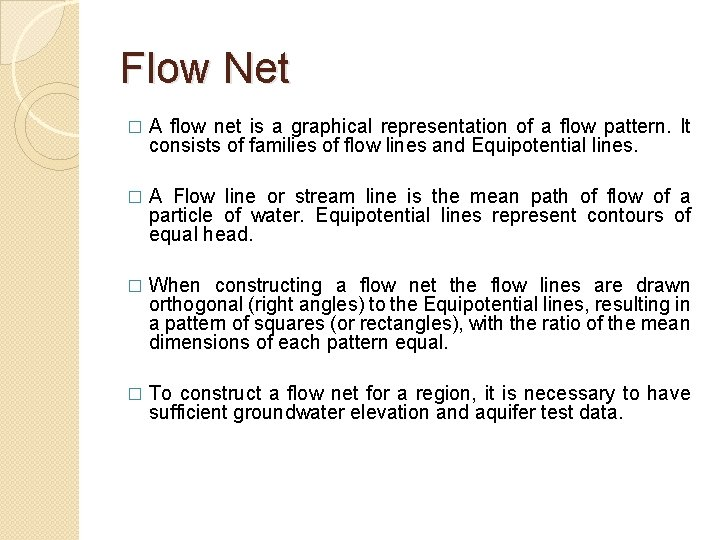Flow Net � A flow net is a graphical representation of a flow pattern. It consists of families of flow lines and Equipotential lines. � A Flow line or stream line is the mean path of flow of a particle of water. Equipotential lines represent contours of equal head. � When constructing a flow net the flow lines are drawn orthogonal (right angles) to the Equipotential lines, resulting in a pattern of squares (or rectangles), with the ratio of the mean dimensions of each pattern equal. � To construct a flow net for a region, it is necessary to have sufficient groundwater elevation and aquifer test data.Assumptions of Flow net Analysis � The aquifer is homogeneous, isotropic and is fully saturated. � The flow field is at steady-state. � The aquifer material and water are incompressible. � Flow is laminar and Darcy’s law is applicable. � Boundary conditions are known. � Stream lines and Equipotential lines intersect at right angles. � Flow quantity between all parts of adjacent streamlines is same. � Energy loss between all pairs of Equipotential lines is same.Flow net analysis � Consider a portion of a flow net. The hydraulic gradient , (Equation 1) � and the constant flow between two adjacent flow lines, (Equation 2) � For unit thickness and for the square of the flow net, the approximation ds ≈ dm � So, Eq. 2 reduces to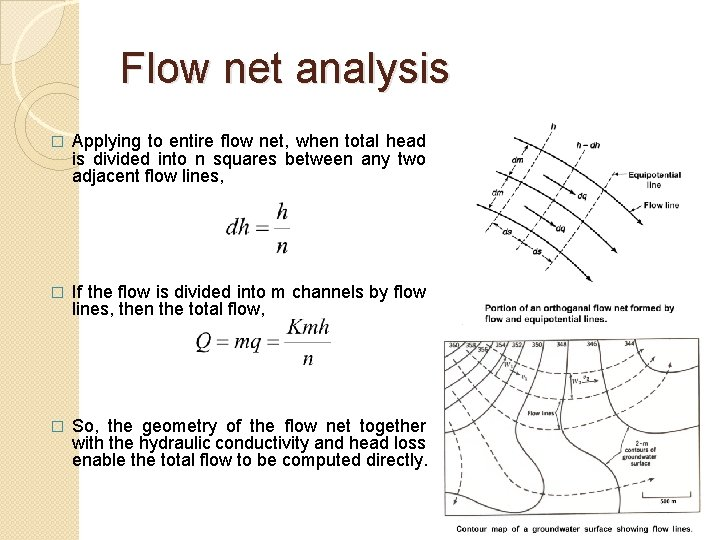Flow net analysis � Applying to entire flow net, when total head is divided into n squares between any two adjacent flow lines, � If the flow is divided into m channels by flow lines, then the total flow, � So, the geometry of the flow net together with the hydraulic conductivity and head loss enable the total flow to be computed directly.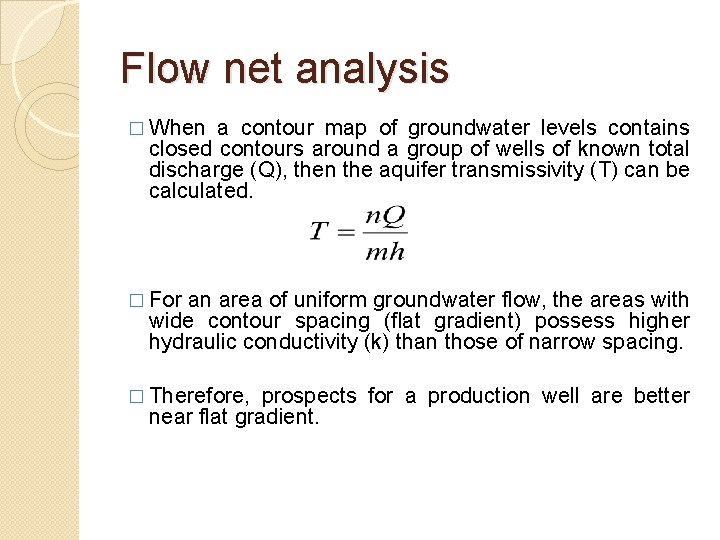Flow net analysis � When a contour map of groundwater levels contains closed contours around a group of wells of known total discharge (Q), then the aquifer transmissivity (T) can be calculated. � For an area of uniform groundwater flow, the areas with wide contour spacing (flat gradient) possess higher hydraulic conductivity (k) than those of narrow spacing. � Therefore, prospects for a production well are better near flat gradient.Advantages of Flow net �Direct calculation of total flow (Q) �Determination of aquifer transmissivity (T) �Direction of flow �Location of new water wells.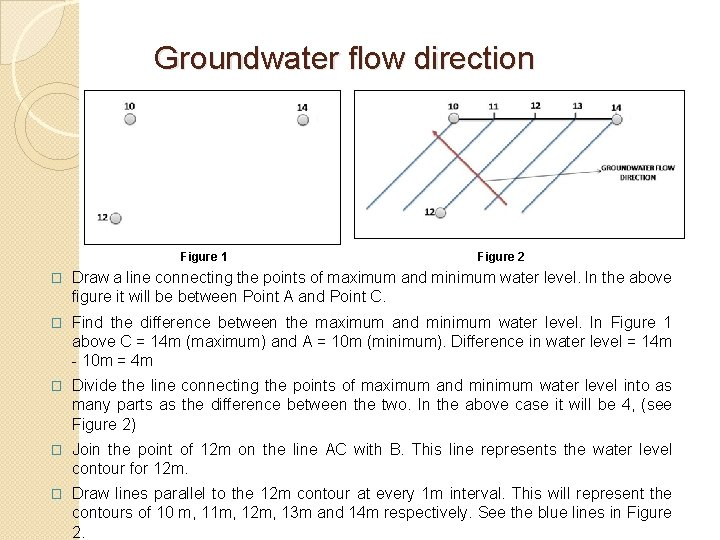Groundwater flow direction Figure 1 Figure 2 � Draw a line connecting the points of maximum and minimum water level. In the above figure it will be between Point A and Point C. � Find the difference between the maximum and minimum water level. In Figure 1 above C = 14 m (maximum) and A = 10 m (minimum). Difference in water level = 14 m - 10 m = 4 m � Divide the line connecting the points of maximum and minimum water level into as many parts as the difference between the two. In the above case it will be 4, (see Figure 2) � Join the point of 12 m on the line AC with B. This line represents the water level contour for 12 m. � Draw lines parallel to the 12 m contour at every 1 m interval. This will represent the contours of 10 m, 11 m, 12 m, 13 m and 14 m respectively. See the blue lines in Figure 2.